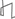Technical Reports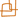Work Products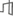Research Abstracts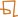Historical Collections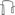Research Abstracts - 2006IntroductionLanguage, Learning, Vision & GraphicsPhysical, Biological & Social SystemsSystemsTheory### Computing Equivalence Classes for Planning

#### Natalia H. Gardiol & Leslie Pack Kaelbling

##### Introduction

In many logical planning domains, the crux of finding a solution often lies in overcoming an overwhelmingly large action space. Consider, as an illustration, the classic blocks world domain: the number of ways to make a stack of a certain height grows exponentially with the number of blocks on the table; and if the outcomes of actions are uncertain, this apparently simple task becomes daunting. We want planning techniques that can deal with large state spaces and large, stochastic action sets, since most compelling, realistic domains have these characteristics.

One way to describe large stochastic domains compactly is to use relational representations. Such a representation allows dynamics of the domain to be expressed in terms of object properties rather than object identities, and, thus, yields a much more compact representation of a domain than the equivalent propositional version can.

Even planning techniques that use relational representations, however often end up operating in a fully-ground state and action space when it comes time to find a solution, since such spaces are conceptually much simpler to handle. In this case, a key insight gives us leverage: often, several action instances produce similar effects. For example, in a blocks world it often does not matter which block is picked up first as long as a stack of blocks is produced in the end. If it were possible to identify under what conditions actions produce equivalent kinds of effects, the planning problem could be simplified by considering a representative action (from each equivalence class) rather than the whole action space.

This work is about taking advantage of structured, relational action representations. We want to identify logically similar effects in order to reduce the effective size of the action space.

##### Relational Envelope-based Planning

The Relational Envelope-based Planning algorithm (REBP)   is well-suited to address planning problems with large underlying spaces. It proceeds in two phases. First, given a domain theory and a problem instance, an initial plan of action is found quickly using classical planning techniques. Classical planning produces a focused search within high-probability sequences of actions, and yields an initial sequence called an \emph{envelope} of states . Second, with additional time, this initial plan can be made more robust by considering deviations from the original envelope. Conditioned on a ground initial state, the number of states we expect to experience on the way to the goal is relatively small; thus, the effectiveness of REBP lies in limiting the state space in which policies searched for to an informative, reachable subset.

A fundamental step, however, is to produce the initial envelope efficiently. When the action space is large, however, this can be hard to do. In this case, a key insight gives us leverage: different ground action instances often produce qualitatively similar effects. For example, in a blocks world it often does not matter which block is picked up first as long as a stack of blocks is produced in the end. If it were possible to identify under what conditions actions produce equivalent kinds of effects, the planning problem could be simplified by considering a representative action (from each equivalence class) rather than the whole action space. The resulting reduction in branching factor can result in huge planning efficiency gains. The figure below shows an example.

In this figure, we have an example domain in which the task is to fly each of the three helicopters onto one of two carriers. In a) is shown a picture of the search tree if we were to enumerate all the ground actions. However, there are only a few qualitatively different states, as seen on the bottom, in c). If we could eliminate distinguishing between actions that produce equivalent states, our search tree would be much more compact (b).##### Finding the initial envelope

Finding a trajectory of states with which to populate the initial envelope involves solving a planning problem from the ground initial state to a state satisfying a logical goal condition.

We represent planning domains in a subset of the {\sc ppddl} language. A problem description contains the following elements: P, a set of logical predicates, denoting the properties and relations that can hold among the finite set of domain objects, O; Z, a set of transition schemas; and T, a set of object types. A schema z in Z, when applied in a state s, produces a set of ground actions, z|s.

To find this plan, we execute heuristic-based search using the FF heuristic. . The algorithm is shown in the figure below. Note steps b) and c), which compute and make use of the reduced action space given by a partition over the actions.##### Equivalence in relational domains

We need to properly define action equivalence in order to execute the steps b) and c) of the planning algorithm in~\figref{algorithm}. To that end, we make the following crucial assumption:

Sufficiency of Object Properties: A domain object's function is determined only by its properties and relations to other objects, and not by its name.

For example, consider a blocks world in which the only two properties are the relation "on/2" and the attribute "color/1". Then if two blocks block14 and block37 are both red, are both on the table, and have nothing on them, they would be considered functionally equivalent. If block37 had another block on top of it, however, it would not be equivalent to block14. Intuitively, two objects are equivalent to each other if they are related in the same way to other objects that are, in turn, equivalent.

Here is the main contribution. We establish that a planning procedure that uses only equivalence-class representatives is complete whenever the original planning procedure, which had access to the whole action space, is complete. We need the following pieces: first, whenever goal is satisfied in a particular state s, then it must be satisfied by any state in s's equivalence class; second, equivalent actions taken from equivalent states produce equivalent successor states. These pieces let us construct an inductive argument to show that, from a given starting state, the successive substitution of one ground action by another in its equivalence class leads us to a state that still satisfies the goal. 

Previous work on object equivalence, or symmetry, has used single, unary relations as a basis for computing similarity . However, we want to study object equivalence when more complex relationships are present. To aid our analysis, we view a relational state description as a graph, called the state relation graph. The nodes in the graph correspond to objects in the domain, and the binary relations between the objects correspond to the edges. For each pair of related nodes, we construct an edge representing the relation. In addition, nodes and edges are labeled with a string (or set of strings). Each node is labeled with the object's type, and each edge is labeled with the relation's name. If an object also participates in a unary relation, we augment its label set with that predicate's name. At present, we consider up to binary relations. In the case of relations with more than two arguments, we would have to consider a hypergraph representation to allow for edges of more than two nodes.

Thus, we can establish equivalence between two states by computing an isomorphism between the state relation graphs. ivalent if they produce equivalent states. However, this requires propagating a state through a transition rule for each calculation. A way to define action equivalence without doing such a propagation is to overload the notion of isomorphism to apply to sentences (of which actions are a special case). The figure below shows an example of this computation.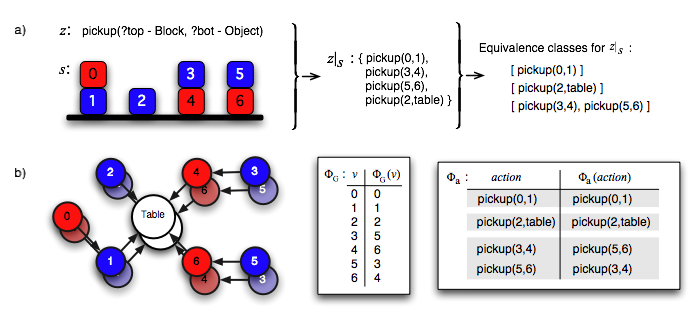The steps involved in computing action equivalence are shown above. In part (a), the instantiation of the pickup operator $z$ in a state $s$ produces four ground actions. In part (b), the state relation graph for s shows we can map blocks 3 and 4 to blocks 5 and 6, respectively. This allows us to map the instantiation of pickup(3,4) to pickup(5,6), and vice-versa. Thus, the four ground actions correspond to three equivalence classes.

We show that any serial plan that exists in the full action space has an equivalent version in the partitioned space.

Taking advantage of structured action representations helps us ignore the distracting complexity and focus instead on the interesting complexity in a problem. We provide a formal basis for computing action equivalence classes that guarantees a complete planning procedure while significantly reducing the branching factor of the search. While our original motivation is the the \rebp\ algorithm, our findings are useful for efficient planning in general.

##### References:

 Gardiol, Natalia H. and Kaelbling, Leslie P.. Envelope-based Planning in Relational MDPs. In Advances in Neural Information Processing 16 (NIPS-2003), Vancouver, 2004.

 Thomas Dean and Leslie Pack Kaelbling and Jak Kirman and Ann Nicholson. Planning Under Time Constraints in Stochastic Domains. 1995.

 J\"org Hoffmann and Bernhard Nebel. The FF Planning System: Fast Plan Generation Through Heuristic Search. 2001.

 Gardiol, Natalia H. and Kaelbling, Leslie Pack. Computing Action Equivalences for Planning Under time constraints. Cambridge, MA, 2005.

 T. Ellman. Abstraction via Approximate Symmetry. In Proceedings of the 13th International Joint Conference on Artificial Intelligence, 1993.

 Fox, Maria and Long, Derek. The Detection and Exploitation of Symmetry in Planning Problems. In 16th International Joint Conference on Artificial Intelligence, 1999.

 Fox, Maria and Long, Derek. Extending the Exploitation of Symmetries in Planning. In AIPS, 2002.Computer Science and Artificial Intelligence Laboratory (CSAIL) The Stata Center, Building 32 - 32 Vassar Street - Cambridge, MA 02139 - USA tel:+1-617-253-0073 - publications@csail.mit.edu